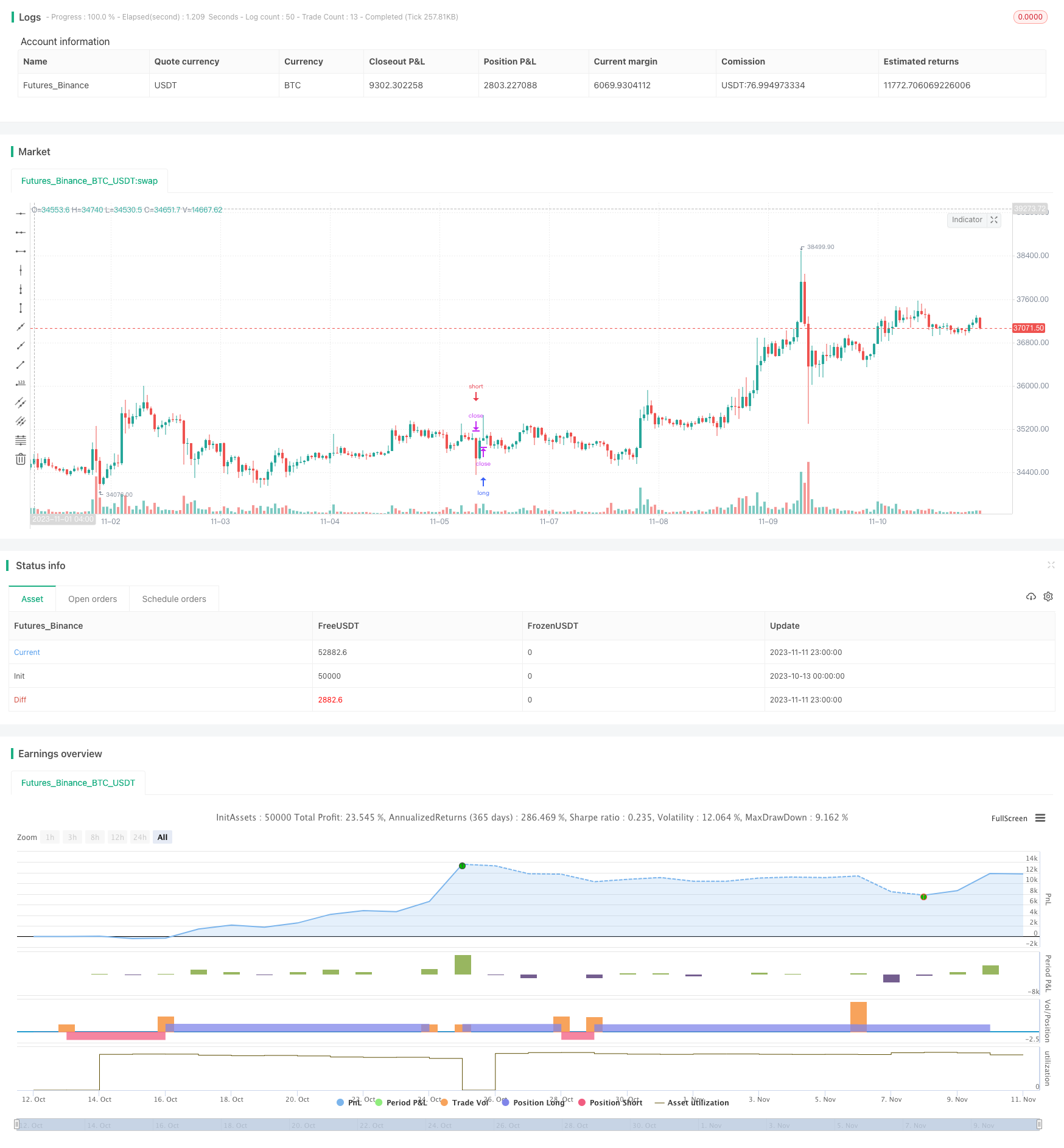# High Probability Breakthrough Trading Strategy Based on Pressure Balance

Author: ChaoZhang, Date: 2023-11-13 11:40:53
Tags:#### Overview

This strategy uses a combination of multiple indicators to determine the trend direction and trading opportunities, adopting a pressure balance approach to increase the winning rate of trades. It mainly utilizes MACD, PSAR and EMA indicators for judgement, and implements stop loss and take profit to achieve effective profitability.

#### Strategy Logic

1. Use EMA to calculate moving average to determine the overall trend direction. Larger EMA value indicates an uptrend, while smaller EMA value indicates a downtrend.

2. Use MACD to calculate the difference between fast and slow moving averages. When the difference is greater than 0, it indicates an uptrend, when less than 0, it indicates a downtrend.

3. Use PSAR to calculate the continuous varying point. When PSAR value is large, it indicates a downtrend, when PSAR value is small, it indicates an uptrend.

4. Combine the above three indicators to determine consistency of the trend. When the judgments of the three indicators are consistent, it represents a clear trend that allows entry trades.

5. Enter trades based on buy and sell criteria, and set stop loss and take profit points. Exit trades when stop loss or take profit conditions are met to realize profits.

6. Specific rules are:

• Buy condition: not in uptrend, MACD histogram < 0, close price > EMA
• Sell condition: in uptrend, MACD histogram > 0, close price < EMA
• Stop loss condition: price hits next PSAR value
• Take profit condition: reaching preset take profit ratio

1. Using multiple indicators to determine the trend improves accuracy.

2. Opening trades based on definite trends identified through pressure balance increases winning rate.

3. Setting stop loss and take profit can limit losses and lock in profits.

4. Clear and systematic trading rules make it suitable for algorithm trading.

5. Parameters can be optimized to adapt to different products and timeframes.

#### Risks of the Strategy

1. Trend judgement may be wrong, resulting in incorrect trade direction.

2. Extreme market moves may generate false signals from the indicators.

3. Stop loss being set too wide, unable to exit timely.

5. Illiquid products cannot fulfill stop loss and take profit plans.

6. Risks can be reduced by optimizing parameters, adjusting stops, and selecting liquid products.

#### Optimization Directions

1. Adjust EMA period to optimize trend accuracy.

2. Tune MACD fast and slow period to improve sensitivity.

3. Optimize stop loss and take profit ratios to find ideal balance.

4. Add other auxiliary indicators to improve entry timing.

5. Select products with good liquidity and large swings.

6. Adjust timeframes to suit different product characteristics.

#### Summary

This strategy integrates multiple indicators for trend analysis and enters trades based on definite trends, with preset stop loss and take profit, which can effectively capture market moves and achieve good returns while ensuring certain profitability. Further improvements on stability and profitability can be achieved through parameter tuning and additional indicators. The clear trading rules make this strategy very suitable for algorithmic trading.

```/*backtest
start: 2023-10-13 00:00:00
end: 2023-11-12 00:00:00
period: 1h
basePeriod: 15m
exchanges: [{"eid":"Futures_Binance","currency":"BTC_USDT"}]
*/

// This source code is subject to the terms of the Mozilla Public License 2.0 at https://mozilla.org/MPL/2.0/

//@version=4
strategy(title = "Crypto Scalper", overlay = true,  pyramiding=1,initial_capital = 100, default_qty_type= strategy.percent_of_equity, default_qty_value = 100, calc_on_order_fills=false, slippage=0,commission_type=strategy.commission.percent,commission_value=0.03)
len = input(60, minval=1, title="Length EMA")
src = input(close, title="Source")
out = ema(src, len)
//
fast_length = input(title="Fast Length MACD", type=input.integer, defval=12)
slow_length = input(title="Slow Length MACD", type=input.integer, defval=26)
signal_length = input(title="Signal Smoothing", type=input.integer, minval = 1, maxval = 50, defval = 9)
sma_source = input(title="Oscillator MA Type MACD", type=input.string, defval="EMA", options=["SMA", "EMA"])
sma_signal = input(title="Signal Line MA Type MACD", type=input.string, defval="EMA", options=["SMA", "EMA"])

// Calculating
fast_ma = sma_source == "SMA" ? sma(src, fast_length) : ema(src, fast_length)
slow_ma = sma_source == "SMA" ? sma(src, slow_length) : ema(src, slow_length)
macd = fast_ma - slow_ma
signal = sma_signal == "SMA" ? sma(macd, signal_length) : ema(macd, signal_length)
hist = macd - signal

start = input(0.02)
increment = input(0.02)
maximum = input(0.2)
var bool uptrend = na
var float EP = na
var float SAR = na
var float AF = start
var float nextBarSAR = na
if bar_index > 0
firstTrendBar = false
SAR := nextBarSAR
if bar_index == 1
float prevSAR = na
float prevEP = na
lowPrev = low
highPrev = high
closeCur = close
closePrev = close
if closeCur > closePrev
uptrend := true
EP := high
prevSAR := lowPrev
prevEP := high
else
uptrend := false
EP := low
prevSAR := highPrev
prevEP := low
firstTrendBar := true
SAR := prevSAR + start * (prevEP - prevSAR)
if uptrend
if SAR > low
firstTrendBar := true
uptrend := false
SAR := max(EP, high)
EP := low
AF := start
else
if SAR < high
firstTrendBar := true
uptrend := true
SAR := min(EP, low)
EP := high
AF := start
if not firstTrendBar
if uptrend
if high > EP
EP := high
AF := min(AF + increment, maximum)
else
if low < EP
EP := low
AF := min(AF + increment, maximum)
if uptrend
SAR := min(SAR, low)
if bar_index > 1
SAR := min(SAR, low)
else
SAR := max(SAR, high)
if bar_index > 1
SAR := max(SAR, high)
nextBarSAR := SAR + AF * (EP - SAR)

tplong=input(0.245, step=0.005)
sllong=input(1.0, step=0.005)
tpshort=input(0.055, step=0.005)
slshort=input(0.03, step=0.005)

if (uptrend and hist >0 and close < out)
strategy.entry("short", strategy.short, stop=nextBarSAR, comment="short")
strategy.exit("short_tp/sl", "short", profit=close * tpshort / syminfo.mintick, loss=close * slshort / syminfo.mintick, comment='SHORT EXIT',  alert_message = 'closeshort')
if (not uptrend and hist <0 and close > out)
strategy.entry("long", strategy.long, stop=nextBarSAR, comment="long")
strategy.exit("short_tp/sl", "long", profit=close * tplong / syminfo.mintick, loss=close * sllong / syminfo.mintick, comment='LONG EXIT',  alert_message = 'closelong')

```

More